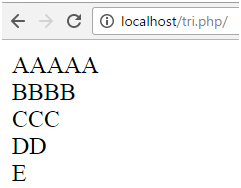Q:

# Alphabet Triangle Method in Php

There are three methods to print the alphabets in a triangle or in a pyramid form.

• range() with for loop
• chr() with for loop
• range() with foreach loop

Logic:

• Two for loops are used.
• First for loop set conditions to print 1 to 5 rows.
• Second for loop set conditions in decreasing order.

## Using range() function

This range function stores values in an array from A to Z. here, we use two for loops.

Example:

``````<?php
\$alpha = range('A', 'Z');
for(\$i=0; \$i<5; \$i++){
for(\$j=5; \$j>\$i; \$j--){
echo \$alpha[\$i];
}
echo "<br>";
}
?>  ``````

Output:## Using chr() function

Here the chr() function returns the value of the ASCII code. The ASCII value of A, B, C, D, E is 65, 66, 67, 68, 69 respectively. Here, also we use two for loops.

Example:

``````<?php
for( \$i=65; \$i<=69; \$i++){
for(\$j=5; \$j>=\$i-64; \$j--){
echo chr(\$i);
}
echo "<br>";
}
?>  ``````

Output:## Using range() function with foreach

In this methods we use foreach loop with range() function. The range() function contain values in an array and returns it with \$char variable. The for loop is used to print the output.

Example:

``````<?php
\$k=1;
foreach(range('A','Z') as \$char){
for(\$i=5; \$i>=\$k; \$i--){
echo \$char;
}
echo "<br>";
\$k=\$k+1;  ``````

Output: